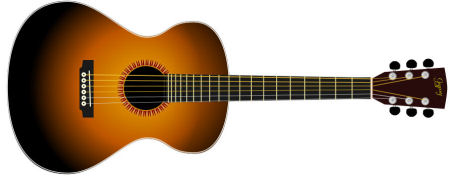Search IntMath
Close

# Vectors

By Murray Bourne, 11 May 2008

Many readers of Interactive Mathematics have asked me to write a chapter on vectors.

Well, your wish is my command and here you go: Vectors.

The chapter starts with an overview of what vectors are and vector notation.

Then the discussion proceeds through one-dimensional vectors, through 2-D vectors (containing a Flash-based aircraft landing activity) and leading to the juicy 3-D vectors stuff like cross product.

There is a brief section on vector calculus, including a discussion on time rate of change of the position vector in elliptical motion, which is used in building Flash-based games.

I added a section at the end on the interesting topic of vector art. Digital artists produce vector graphics in image editing programs like Photoshop or the open source Inkscape. The advantages are many, ranging from scalability and small file sizes.

This guitar is an example of vector art.I'll be adding more discussion, examples and exercises into the chapter when I get the chance − hopefully soon!

Let me know what you think about the Vectors chapter, including your suggestions for things you'd like to see added in there.

### Comment Preview

HTML: You can use simple tags like <b>, <a href="...">, etc.

To enter math, you can can either:

1. Use simple calculator-like input in the following format (surround your math in backticks, or qq on tablet or phone):
a^2 = sqrt(b^2 + c^2)
(See more on ASCIIMath syntax); or
2. Use simple LaTeX in the following format. Surround your math with $$ and $$.
$$\int g dx = \sqrt{\frac{a}{b}}$$
(This is standard simple LaTeX.)

NOTE: You can mix both types of math entry in your comment.